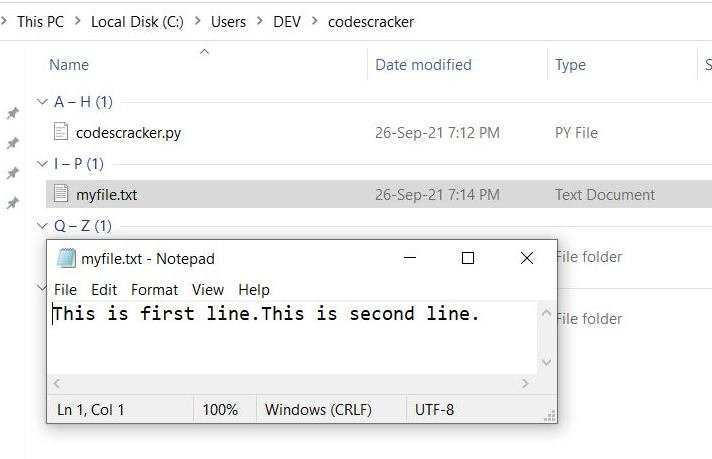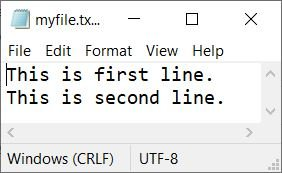# Python writelines() Function

The writelines() function in Python, used to write multiple lines of text or string to a file at once. The lines are in the form of elements of a list. Therefore, we can use string as element of a list to insert multiple strings to a file using writelines() function at once.

## Python writelines() Syntax

The syntax of writelines() function in Python is:

`fh.writelines(list_or_sequence)`

where fh indicates to the file handler or file object and list_or_sequence refers to a list or a sequence.

## Python writelines() Example

Now let's create an example program, demonstrating the writelines() function of Python:

```file_handler = open("myfile.txt", "w")
file_handler.writelines(["This is first line.", "This is second line."])
file_handler.close()
print("The content is written in the file.")```

The snapshot given below shows the sample run of above Python program:Now if you open your current directory. The directory where you're saving your Python's source code. Then you'll see, a file named myfile.txt is created with This is first line.This is second line. as its content. Here is the snapshot of the current directory with opened this newly created file using above program's sample run:See, the content of the file. Both lines are written in single line. This is because, I've not inserted a newline before writing the second sentence. Therefore we need to place \n when we want to insert a newline. Therefore, using writelines(), multiple strings (paragraphs or whatever you say) can be written in the file.

Here is the modified version of previous program, shows how to insert a new line or how to write content in a file, in line by line manner:

```file_handler = open("myfile.txt", "w")
file_handler.writelines(["This is first line.\n", "This is second line."])
file_handler.close()
print("The content is written in the file.")```

This program produces same output as of previous program. But if you open the file named myfile.txt from current directory. Then this time, the two sentences are written in two lines as shown in the snapshot given below:Because, I've used w opening mode, therefore the content of file gets overwritten. Or the previous content gets deleted and the new content gets written.

Now let's modify the program and create a new one that will get the lines or texts from user at run-time of the program:

```print("Enter File's Name: ", end="")
file_name = input()
print("\n1. Write.")
print("2. Append.")
print("Enter Your Choice (1 or 2): ", end="")
choice = int(input())
if choice == 1:
file_handler = open(file_name, "w")
print("\nHow many lines to write: ", end="")
no_of_lines = int(input())
print("Enter", no_of_lines, "lines: ", end="")
my_lines = list()
for i in range(no_of_lines):
line = input()
line = line + "\n"
my_lines.append(line)
file_handler.writelines(my_lines)
file_handler.close()
file_handler = open(file_name, "r")
print("\n----Content of File----")
file_handler.close()
elif choice == 2:
file_handler = open(file_name, "a")
print("\nHow many lines to append: ", end="")
no_of_lines = int(input())
print("Enter", no_of_lines, "lines: ", end="")
my_lines = list()
for i in range(no_of_lines):
line = input()
line = line + "\n"
my_lines.append(line)
file_handler.writelines(my_lines)
file_handler.close()
file_handler = open(file_name, "r")
print("\n----Content of File----")
file_handler.close()
else:
print("\nWrong choice!")```

Here is its sample run with user input codescracker.txt as name of file, 1 as choice, 3 as number of lines to write, and I'm first line. as first element, I'm second line. as second element, and I'm third line. as third element:Python Online Test

« Previous Function Next Function »